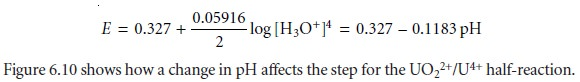Home | | Modern Analytical Chemistry | Ladder Diagram for Oxidationâ€“Reduction Equilibria

# Ladder Diagram for Oxidationâ€“Reduction Equilibria

Ladder diagrams can also be used to evaluate equilibrium reactions in redox systems.

Ladder diagrams can also be used to evaluate equilibrium reactions in redox sys- tems. Figure 6.9 shows a typical ladder diagram for two half-reactions in which the scale is the electrochemical potential, E. Areas of predominance are defined by the Nernst equation. Using the Fe3+/Fe2+ half-reaction as an example, we writeFor potentials more positive than the standard-state potential, the predominate species is Fe3+, whereas Fe2+ predominates for potentials more negative than EÂ°. When coupled with the step for the Sn4+/Sn2+ half-reaction, we see that Sn2+ can be used to reduce Fe3+. If an excess of Sn2+ is added, the potential of the resulting solu- tion will be near +0.154 V.

Using standard-state potentials to construct a ladder diagram can present problems if solutes are not at their standard-state concentra- tions. Because the concentrations of the reduced and oxidized species are in a logarithmic term, deviations from standard-state concentra- tions can usually be ignored if the steps being compared are separated by at least 0.3 V.1b A trickier problem occurs when a half-reactionâ€™s po- tential is affected by the concentration of another species. For example, the potential for the following half-reactiondepends on the pH of the solution. To define areas of predominance in this case, we begin with the Nernst equationand factor out the concentration of H3O+.From this equation we see that the areas of predominance for UO22+ and U4+ are defined by a step whose potential isStudy Material, Lecturing Notes, Assignment, Reference, Wiki description explanation, brief detail
Modern Analytical Chemistry: Equilibrium Chemistry : Ladder Diagram for Oxidationâ€“Reduction Equilibria |